# HSPT Math : Concepts

## Example Questions

### Example Question #3 : How To Find The Surface Area Of A Sphere

A sphere has its center at the origin.  A point on its surface is found on the x-y axis at (6,8).  In square units, what is the surface area of this sphere?

200π

400π

40π

(400/3)π

400π

Explanation:

To find the surface area, we must first find the radius.  Based on our description, this passes from (0,0) to (6,8).  This can be found using the distance formula:

62 + 82 = r2; r2 = 36 + 64; r2 = 100; r = 10

It should be noted that you could have quickly figured this out by seeing that (6,8) is the hypotenuse of a 6-8-10 triangle (which is a multiple of the "easy" 3-4-5).

The rest is easy.  The surface area of the sphere is defined by:

A = 4πr2 = 4 * 100 * π = 400π

### Example Question #1522 : Concepts

A sphere is perfectly contained within a cube that has a surface area of 726 square units. In square units, what is the surface area of the sphere?

121π

484π

11π

30.25π

121π

Explanation:

To begin, we must determine the dimensions of the cube. To do this, recall that the surface area of a cube is made up of six squares and is thus defined as: A = 6s2, where s is one of the sides of the cube. For our data, this gives us:

726 = 6s2; 121 = s2; s = 11

Now, if the sphere is contained within the cube, that means that 11 represents the diameter of the sphere. Therefore, the radius of the sphere is 5.5 units. The surface area of a sphere is defined as: A = 4πr2. For our data, that would be:

A = 4π * 5.52 = 30.25 * 4 * π = 121π

### Example Question #1523 : Concepts

The area of a circle with radius 4 divided by the surface area of a sphere with radius 2 is equal to:

1

0.5

π

3

2

1

Explanation:

The surface area of a sphere is 4πr2. The area of a circle is πr2. 16/16 is equal to 1.

### Example Question #1524 : Concepts

What is the ratio of the surface area of a cube to the surface area of a sphere inscribed within it?

3/π

6/π

2π

4/π

π/3

6/π

Explanation:

Let's call the radius of the sphere r. The formula for the surface area of a sphere (A) is given below:

A = 4πr2

Because the sphere is inscribed inside the cube, the diameter of the sphere is equal to the side length of the cube. Because the diameter is twice the length of the radius, the diameter of the sphere is 2r. This means that the side length of the cube is also 2r

The surface area for a cube is given by the following formula, where s represents the length of each side of the cube:

surface area of cube = 6s2

The formula for surface area of a cube comes from the fact that each face of the cube has an area of s2, and there are 6 faces total on a cube.

Since we already determined that the side length of the cube is the same as 2r, we can replace s with 2r.

surface area of cube = 6(2r)= 6(2r)(2r) = 24r2.

We are asked to find the ratio of the surface area of the cube to the surface area of the sphere. This means we must divide the surface area of the cube by the surface area of the sphere.

ratio = (24r2)/(4πr2)

The rterm cancels in the numerator and denominator. Also, 24/4 simplifes to 6.

ratio = (24r2)/(4πr2) = 6/π

### Example Question #683 : Geometry

What is the surface area of a hemisphere with a diameter of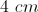?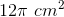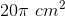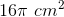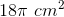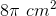Explanation:

A hemisphere is half of a sphere.  The surface area is broken into two parts:  the spherical part and the circular base.

The surface area of a sphere is given by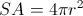.

So the surface area of the spherical part of a hemisphere is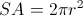The area of the circular base is given by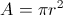.  The radius to use is half the diameter, or 2 cm.

### Example Question #21 : How To Find Surface Area

Find the surface area of a sphere with a radius of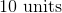.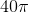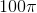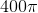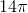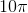Explanation:

Write the surface area formula for a sphere.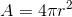Substitute the value of the radius.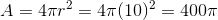### Example Question #341 : Geometry

Find the surface area of a cube with a side length of.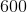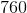Explanation:

Write the formula for the surface area of a cube.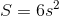Substitute the length.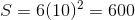### Example Question #1521 : Concepts

What is the surface area of a cube with a side length of three?Explanation:

Write the formula for the surface area of a cube.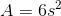Substitute the side length into the equation.Simplify the square inside the parentheses and multiply.### Example Question #2121 : Hspt Mathematics

Find the surface area of a cube with side length.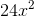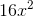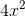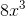Explanation:

To solve, simply use the following formula for the surface area of a cube.

Thus,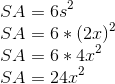### Example Question #1522 : Concepts

If a cube has an area ofon one of its sides, what is the total surface area?Explanation:

A cube hassides that have equal length edges and also equal side areas.

To find the total surface area, you just need to multiple the side area () bywhich is,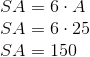.

### All HSPT Math Resources